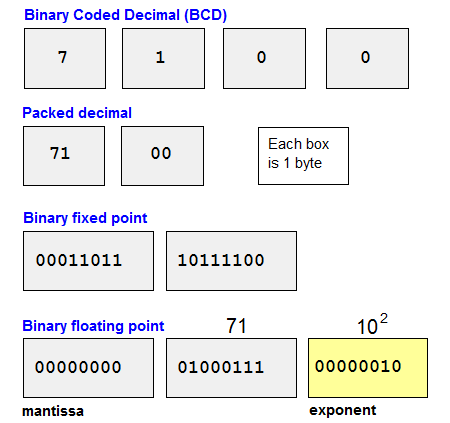# floating point

(redirected from Floating point value)
Also found in: Dictionary, Acronyms.

## floating point

A method for storing and calculating numbers in which the decimal points do not line up as in fixed point numbers. The significant digits are stored as a unit called the "mantissa," and the location of the radix point (decimal point in base 10) is stored in a separate unit called the "exponent." Floating point methods are used for calculating a large range of numbers quickly.

Floating point operations can be implemented in software or in a floating point unit (FPU), which may be a separate "math coprocessor" chip or a circuit in the CPU. See math coprocessor, binary numbers and NaN.

```FLOATING POINT EXAMPLESMantissa  Exponent  Value

71        0           71
71        1          710
71        2         7100
71        -1           7.1
```

How Numbers Are StoredFloating point is one of four primary ways numbers are stored in the computer.

## Floating Point

a form of representation of numbers in a digital computer, with variable position of the point that separates the whole part of the number from the fraction. A floating point corresponds to the normal or semilogarithmic form of representation of numbers. For example, the numbers 5671.31 (a decimal number) and 1101.1 (a binary number) are represented in normalized form as follows: 0.567131 x 10+4 and 0.11011 x 10+100. The range of numbers that can be represented is much broader in a digital computer with a floating point than in one with a fixed point.

A floating point improves the accuracy of calculations and excludes large coefficients for most problems, thus facilitating the process of programming and problem preparation, but operations involving numbers with a floating point are more labor-intensive and digital computers with a floating point are structurally more intricate than are digital computers with a fixed point. The representation of numbers with a floating point is used in most Soviet digital computers, such as the Minsk-22, Ural-14, BESM-4, BESM-6, and M-220.

Site: Follow: Share:
Open / Close